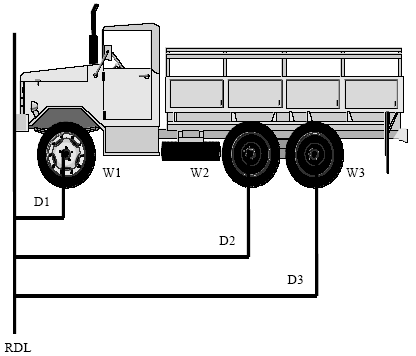#### Weight and Balance Equations and Calculator For Trailers

This calculator will determine the Weigh and Balance of a trailer or similar application. Units used are in, lbs or mm and Newton's perspective. At the bottom of the webpages, there are several weight and balance application example's.Conversion:

1 in = 25.4 mm
1 lbs = 4.44822 Newton's

Of course you can use kilograms in place of newton's.
1 kg = 9.80665 Newton'sor 2.2046 lbs
(Whatever makes you happy).

To determine the center of balance (CB) and weight locations following the following procedure.

1. Use the following formula to compute the CB location of vehicles. Multiply weight by distance of each axle from the reference line (in inches), and then divide the total results by the vehicle gross weight. The resulting figure is the number of inches to be measured aft from the reference line to the CB of the vehicle.

Center of Balance Formula
Wl -- Front axle weight.
W2 -- Rear axle weight.
DI -- Distance from RDL to front axle or center of articulated tandem axle
D2 -- Distance from RDL to rear axle or center of articulated tandem axle.

2. The vehicle CB is computed to the nearest whole inch. Any answer with a fraction of .5 or higher is increased to next higher number. If .4 or less, drop the number.

Calculation example #1

Step 1. Weigh all axles individually.
Step 2. Mark weight above each individual axle.
Step 3. Establish the RDL at the forward edge of the vehicle.
Step 4. Measure all distances from RDL to center of each individual axle.
Step 5. Distance multiplied by weight equals a moment.

Example of basic formula for determining the CB.

(Dl x W1) + (D2 x W2)
GROSS WEIGHT = CB from RDLD I from RDL (from forward edge) to center of front axle = 20"
W1 front axle weight = 2,870 lbs
D2 from RDL (from forward edge) to center of rear axle - 150"
W2 rear axle weight = 2,550 lbs

 20" x 2,870 = 57,400 moment 150" x 2.550 = 382,500 moment 439,900 total moment

W1 (2,870 lbs) + W2 (2550 lbs) = Gross Weight (5,420 lbs)
Total Moment (439,900) divided by Gross Weight = CB (81" from RDL)
[(D1 (20") x W1 (2,870 lbs)] +[ (D2 (150" x W2 (2,550 lbs)]
Gross Weight (5,420 lbs) = CB (81" from RDL)

Calculation example #2[D1 (15") x W1 (250 lbs)] + [D2 (102") x W2 (2,250 lbs)]
W1 (250 lbs) + W2 (2,250 lbs) = Gross Weight (2,500 lbs) = CB (93" from RDL)

Calculation example #3[D1 (70") x W1 (12,500 lbs)] + [D2 (222") x W2 (12,900 lbs)] + [D3 (276") x W3 (12,700 lbs)]
W1 (12,500 lbs) + W2 (12,900 lbs) + W3 (12,700 lbs) = Gross weight (38,100 lbs) = CB (190" from RDL)

Related:

• Simple Machine Levers Equations and Calculation